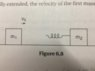# When the spring is maximally extended, find v_1f

• JessicaHelena

## Homework Statement

A mass m_1, initially moving at a speed v_0, collides with and sticks to a spring attached to a second, initially stationary mass m_2. The two masses continue to move to the right on a frictionless surface as the length of the spring oscillates. At the instant that the spring is maximally extended, the velocity of the first mass is...?

p_i = p_f
E_i = E_f

## The Attempt at a Solution

Initially, there's only m_1 moving, so p_i = m_1v_0.
When the masses collide and stick together, assuming the spring to be massless, they will travel at a final velocity v_f together (so v_f is the velocity of the center of mass), and p_f = (m_1+m_2)*v_f.

Equating p_i and p_f, we get that v_f = m_1v_0/(m_1+m_2).

However, I'm not sure how to carry on from here, with the spring oscillating. This sounds like m_2 might have a greater velocity and m_1 a little less at some times and other times m_1 might have more velocity than m_2 does, but how can I figure this out definitely?

#### Attachments

•IMG_0213.jpg
23 KB · Views: 265